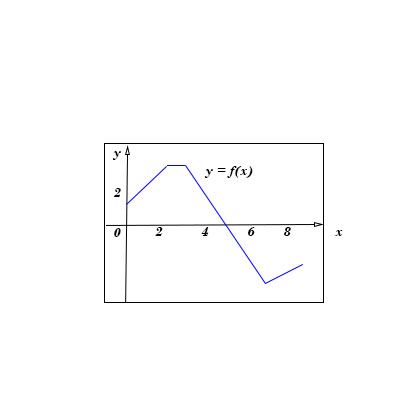# The graph of f is shown. Evaluate each integral by interpreting it in terms of areas. a. \int_0^2...

## Question:

The graph of {eq}f {/eq} is shown. Evaluate each integral by interpreting it in terms of areas.

a. {eq}\int_0^2 f(x) dx {/eq}

b. {eq}\int_0^5 f(x) dx {/eq}

c. {eq}\int_5^7 f(x) dx {/eq}

d. {eq}\int_0^9 f(x) dx {/eq}## Definite Integrals as Areas

The definite integral of a function {eq}\displaystyle f(x) {/eq} over an interval {eq}\displaystyle [a,b] {/eq}

is given as {eq}\displaystyle \int_a^b f(x)dx {/eq} and is equal to the signed area between the graph of {eq}\displaystyle f(x) {/eq} and the x-axis, between {eq}\displaystyle a {/eq} and {eq}\displaystyle b. {/eq}

If the graph is bellow the x-axis, then the area will be taken negative, while if the graph is above the x-axis, the area will be taken positive.

(a) Because the graph of {eq}\displaystyle f(x) {/eq} between {eq}\displaystyle 0 \text{ and } 2 {/eq} is above the x-axis, {eq}\displaystyle \int_0^{2} f(x)dx {/eq} is the area bellow the graph,

which is the area of the trapezoid with the vertical bases of lengths 2 and 4 and the horizontal height of length 2.

Therefore {eq}\displaystyle \int_0^{2} f(x)dx =\frac{(2+4)\cdot 2}{2}=\boxed{6}. {/eq}

b) The graph of {eq}\displaystyle f(x) {/eq} between {eq}\displaystyle 0 \text{ and } 5 {/eq} is above the x-axis,

{eq}\displaystyle \int_0^{5} f(x)dx {/eq} is the area bellow the graph, which is the area of the trapezoid from part (a),

and the trapezoid with the horizontal bases of lengths 1 and 3 and the vertical height of length 4.

Therefore {eq}\displaystyle \int_0^{5} f(x)dx =\int_0^{2} f(x)dx+\int_{2}^{5} f(x)dx =6+\frac{(1+3)\cdot 4}{2}=6+8=\boxed{14}. {/eq}

c) The graph of {eq}\displaystyle f(x) {/eq} between {eq}\displaystyle 5 \text{ and } 7 {/eq} is bellow the x-axis, and {eq}\displaystyle \int_{5}^{7} f(x)\ dx {/eq} is the negative area of the right triangle of sides 2 and 4.

Therefore {eq}\displaystyle \int_{5}^{7} f(x)\ dx =-\frac{2\cdot 4}{2}=\boxed{-4}. {/eq}

d) {eq}\displaystyle \int_0^{9} f(x)\ dx =\int_{0}^{5}f(x)\ dx+\int_{5}^{7} f(x)\ dx+\int_{7}^{9}f(x\ )dx. {/eq}

The graph of {eq}\displaystyle f(x) {/eq} between {eq}\displaystyle 7 \text{ and } 9 {/eq} is bellow the x-axis, therefore {eq}\displaystyle \int_{7}^{9} f(x)\ dx {/eq} the negative area of the trapezoid with the vertical bases of lengths 4 and 2 and the horizontal height of length 2.

Therefore {eq}\displaystyle \int_0^{9} f(x)dx =6+14-4 -\frac{(4+2)\cdot 2}{2}=\boxed{10}. {/eq}